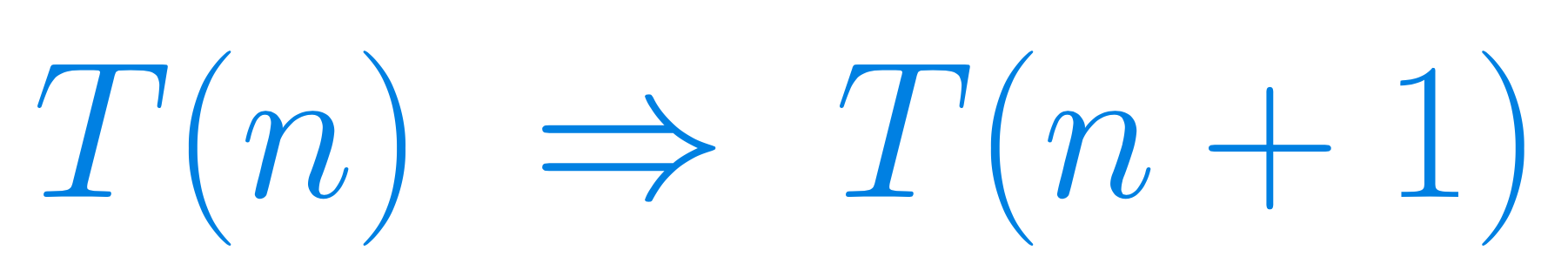Select Page

# Proofs by induction

#### A 2 hour free course on proofs by induction

This is a two hour long course about mathematical proofs by induction.#### Prerequisites

High-school maths, mainly arithmetic.

#### Curriculum

This course concentrates on just one topic, proofs by induction, which is usually introduced in high-school and then comes back in various courses on college or university level.

# Proofs by induction

h

## Get the outline

A detailed list of the lectures can be found in a PDF outline by clicking this panel.## Get Proofs by induction

This is a free course. Just click this panel to be taken to the download page on Udemy.

## Course Objectives & Outcomes

Z
How to conduct proofs by induction and in what circumstances we should use them.
Z
Prove (by induction) some statements about divisibility of natural numbers.
Z
Prove (by induction) some simple inequalities holding for natural numbers.
Z
Prove (by induction) some formulas holding for natural numbers.
Z
Prove (by induction) explicit formulas for sequences defined in a recursive way.
Z
You will also get information about more advanced examples of proofs by induction.
Z
You will get a short explanation how to use the symbols Sigma and Pi for sums and products.